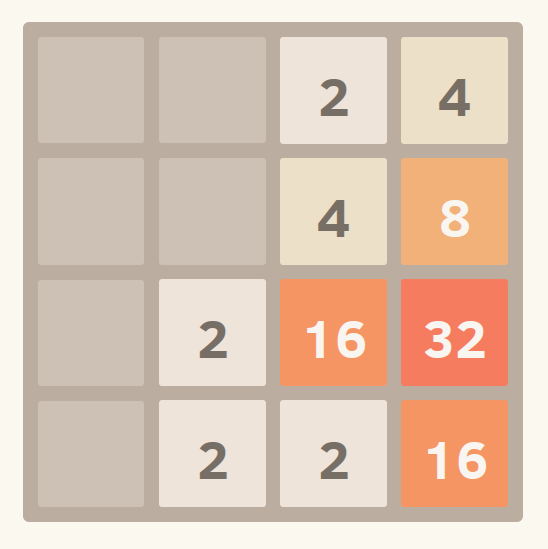# 4096 II

Chris decided to code a game called 4096 which he believes is the next big thing. The game is relatively simple. You have a $4\times 4$ grid that contains integers that are all powers of 2. Tiles slide as far as possible in the chosen direction until they are stopped by either another tile or the edge of the grid. If two tiles of the same number collide while moving, they will merge into a tile with the total value of the two tiles that collided. The resulting tile cannot merge with another tile again in the same move.

The possible moves are $L,R,U,D$ which means swipe to the left, right, up and down respectively. Eventually, there will be some random number pops out and might replace the old tile.Wikipedia

This file contains 1000 lines. Each lines is an operation either :

• L : swipe to the left
• R : swipe to the right
• U : swipe up
• D : swipe down
• P x y v : replace cell $(x,y)$ with number $v$. It is guaranteed that $v$ is a power of 2.

What is the sum of all numbers in the grid after all operations are executed?

Details and Assumptions

The top row from left to right are cells $(1,1), (1,2), (1,3), (1,4)$.

×